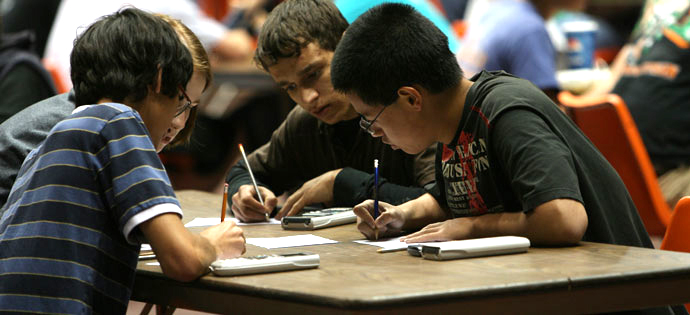# Brilliant's Mathematical Contest!

Logic Level 5In a Mathematics Contest conducted by Brilliant, only the following facts are known to its staff:

• The number of problems asked in the contest was ${n \geq 4}$.

• Each problem was solved by exactly four contestants.

• For each pair of problems, there is exactly one contestant who solved both the problems.

Assuming that the number of contestants is greater than or equal to ${4n}$, help the staff find the minimum value of ${n}$ for which there always exists a contestant who solved all the problems.

###### Image Credit: MissiouriState.edu.
×

Problem Loading...

Note Loading...

Set Loading...